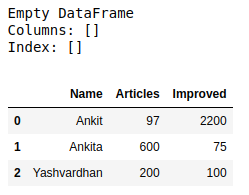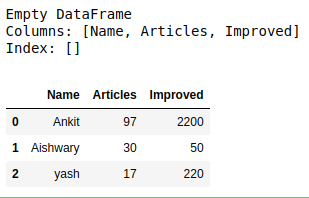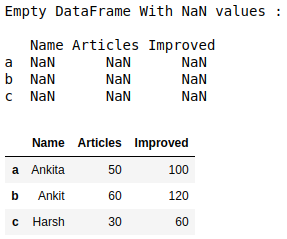How to create an empty DataFrame and append rows & columns to it in Pandas?

• Last Updated : 02 Jul, 2020

Let’s discuss how to create an empty DataFrame and append rows & columns to it in Pandas. There are multiple ways in which we can do this task.

Method #1: Create a complete empty DataFrame without any column name or indices and then appending columns one by one to it.

 # import pandas library as pdimport pandas as pd  # create an Empty DataFrame objectdf = pd.DataFrame()  print(df)  # append columns to an empty DataFramedf['Name'] = ['Ankit', 'Ankita', 'Yashvardhan']df['Articles'] = [97, 600, 200]df['Improved'] = [2200, 75, 100]  df

Output:Method #2: Create an empty DataFrame with columns name only then appending rows one by one to it using append() method.

 # import pandas library as pdimport pandas as pd  # create an Empty DataFrame# object With column names onlydf = pd.DataFrame(columns = ['Name', 'Articles', 'Improved'])print(df)  # append rows to an empty DataFramedf = df.append({'Name' : 'Ankit', 'Articles' : 97, 'Improved' : 2200},                 ignore_index = True)df = df.append({'Name' : 'Aishwary', 'Articles' : 30, 'Improved' : 50},                ignore_index = True)df = df.append({'Name' : 'yash', 'Articles' : 17, 'Improved' : 220},               ignore_index = True)  df

Output:Method #3: Create an empty DataFrame with a column name and indices and then appending rows one by one to it using loc[] method.

 # import pandas library as pdimport pandas as pd  # create an Empty DataFrame object With# column names and indices df = pd.DataFrame(columns = ['Name', 'Articles', 'Improved'],                    index = ['a', 'b', 'c'])  print("Empty DataFrame With NaN values : \n\n", df)  # adding rows to an empty # dataframe at existing indexdf.loc['a'] = ['Ankita', 50, 100]df.loc['b'] = ['Ankit', 60, 120]df.loc['c'] = ['Harsh', 30, 60]  df

Output:Attention geek! Strengthen your foundations with the Python Programming Foundation Course and learn the basics.

To begin with, your interview preparations Enhance your Data Structures concepts with the Python DS Course. And to begin with your Machine Learning Journey, join the Machine Learning - Basic Level Course

My Personal Notes arrow_drop_up